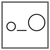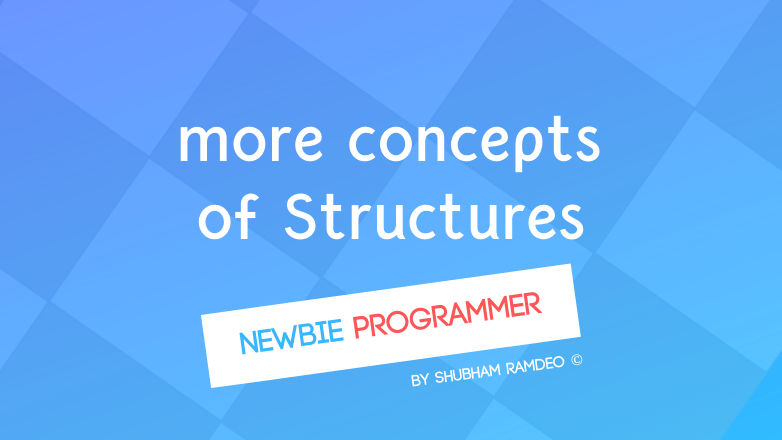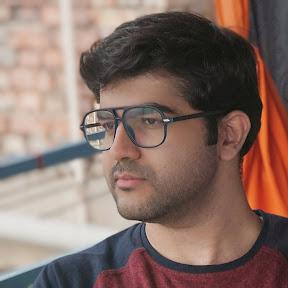Fuddlepixel coz tech is fun();

# Structure more concepts

Let us discuss more concepts about structure. How to use them as functions, macros, nesting them, and more uses to make them as real objects!

By Shubham Ramdeo
24 April 2016 · 4 mins read
# `c`  `newbie`That’s how we play with structs!Welcome to the Newbie Programmer Series. In the last part, we have discussed structures and got a good introduction about them. We learned how to make them and use them as a real world object. In this part, we will discuss more advanced concepts about structure like using them with arrays and functions etc. So if you are new to this series, please go to index (click here) and check out all the previous parts so that you can easily understand what we are discussing down below.

### Arrays of Structure

Let’s get back to where we ended in the last part.

``````typedef struct person {
char name;
int age;
float income;
int child;
};
person john, max;
john.income = 40000;
max.income = 2 * john.income;
``````

In the above ‘person’ structure, we have used arrays inside it. The ‘name’ string. We can say :

``````person john;
john.name = "John The Great";
``````

Now what would be ‘john.name’ ? It’s ‘n’. So we can do anything with that. You can create any data table inside the structure. All the topics that we have a discussed with arrays can be easily implemented inside structures too. Just use ‘.’ (Dot) to access them.

We can also make arrays of structure. For example, in the above code, we have created two persons. John and Max. What if we want to create 100 persons ? Like 100 employees in a company? We can make an array of 100 persons. Like this :

``````person employee;
``````

Now Each of these 100 employees have the properties of person. If we choose that our Mr. John has a code number 44 then we can redefine his properties as :

``````employee.name = "John";
employee.age = 32;
employee.income = 40000;
``````

It means that employee number 44 has name “John”, age 32 and income 40000.

### Nested Structure

We can also use structure inside structure, or what we should call now as “ nested structure “. For example :

``````struct {
int age;
int income;
struct {
int price;
int weight;
} car;
int birthyear;
} john;
``````

In this structure above, we have created another structure called car. Now we can do stuff like this :

``````john.age = 45;
john.car.price = 100000;
``````

You can also make these structures separately and then combine when needed like :

``````typedef struct {
int price;
int weight;
} vehicle;

typedef struct {
int age;
int income;
vehicle car;
int birthyear;
} person;

person john;

john.car.price = 100000;
``````

Same result. But the code looks more realistic.

I think I have used typedef too much. Please take a look the last post again to get it right!

### A little geometry

My favorite application about structure is how they can be use beautifully with geometry. For example, how you represent a point on a graph paper? Such as in this picture below, the Red Point?

[caption id=”attachment_476” align=”aligncenter” width=”150”]See the red point located at (2 ,3)[/caption]

In this picture above, the horizontal line, on which ‘X’ is written, is called X axis, and the vertical line, on which ‘Y’ is written is called Y axis. They are scaled with numbers, negative to positive. Whatever point we want to locate, check out it’s interaction with the X and Y axis and write it as (X , Y). So here, location of the point is (2, 3).

How can we represent such a point in C language? By using structs! And we will use typedef to use them as real objects. I mean as a variable type.

``````typedef struct {
int x;
int y;
} point;
``````

Now see the magic :

``````point p1, p2;

p1.x = 2;
p1.y = 3;

p2.x = 5;
p2.y = 7;
``````

Here we have created two points, p1(2,3) and p2(5,7).

### Structure with Macros

You see we have assigned each value individually, (p1.x=2…). A better idea is to use a MACRO. Yes we can use structs with MACRO too. Such as :

``````#define ADDPOINT(NAME, X, Y) point NAME; NAME.x=X; NAME.y=Y;
``````

Now we can simply do like this :

``````ADDPOINT (P, 2, 3);
``````

This will create our point P with (2, 3) coordinates.

### Structure with Functions

Just as we have used structure with MACRO, we can use them with functions too. Let take this coordinate geometry math further and learn one more thing. Using structure with functions. Declaring the point structure outside the main function, (making it global, see functions) we can make a function like :

``````struct point {
int x;
int y;
};
sayPoint(struct point p)
{
printf("The point has X axis %d and Y axis %d\n", p.x, p.y);
}
``````

Now from the main function :

``````struct point A;
A.x=2;
A.y=3;
sayPoint(A);
``````

Will produce the output :

``````The point has X axis 2 and Y axis 3
``````

I think it’s enough for today. In the next post, we will discuss some more practical applications and will end the structure topic. So stay connected :)

##### Update me weekly ✉

Subscribe below and we’ll send you a weekly email summary of all new Code tutorials. Never miss out on learning about the next big thing.

#### Shubham RamdeoShubham Ramdeo is the founder of Fuddlepixel. He is a geek, a writer, involved with many technical and humanity projects.

Meet me!

#### Recent#### Related

`c`

`newbie`

Top